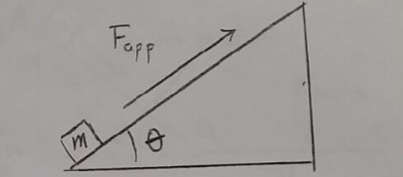Problem: Consider the arrangement shown in the figure. A box of mass m = 5.00 kg is pushed up a slope of inclination angle θ = 25° by an applied force Fapp. The coefficients of static and kinetic friction between the box and the inclined plane are μs = 0.30 and μk = 0.20 respectively. Suppose now that Fapp is increased to the value Fapp = F2 = 40.0 N. Calculate the resulting acceleration of the box up the plane.

FREE Expert Solution
82% (491 ratings)
Problem Details

Consider the arrangement shown in the figure. A box of mass m = 5.00 kg is pushed up a slope of inclination angle θ = 25° by an applied force Fapp. The coefficients of static and kinetic friction between the box and the inclined plane are μs = 0.30 and μk = 0.20 respectively. Suppose now that Fapp is increased to the value Fapp = F2 = 40.0 N. Calculate the resulting acceleration of the box up the plane.